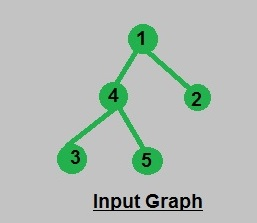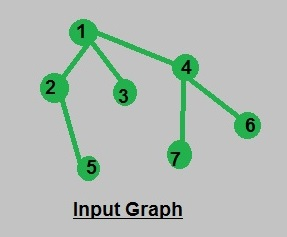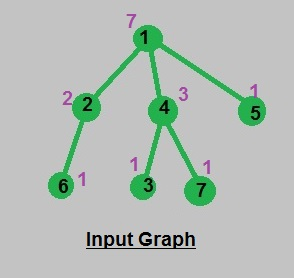GFG App
Open AppBrowser
Continue

# Calculate number of nodes in all subtrees | Using DFS

Given a tree in the form of adjacency list we have to calculate the number of nodes in the subtree of each node while calculating the number of nodes in the subtree of a particular node that node will also be added as a node in subtree hence the number of nodes in subtree of leaves is 1.

Examples:

`Input : Consider the Graph mentioned below:````Output : Nodes in subtree of 1 : 5
Nodes in subtree of 2 : 1
Nodes in subtree of 3 : 1
Nodes in subtree of 4 : 3
Nodes in subtree of 5 : 1

Input : Consider the Graph mentioned below:``````Output : Nodes in subtree of 1 : 7
Nodes in subtree of 2 : 2
Nodes in subtree of 3 : 1
Nodes in subtree of 4 : 3
Nodes in subtree of 5 : 1
Nodes in subtree of 6 : 1
Nodes in subtree of 7 : 1```

Explanation: First we should calculate value count[s] : the number of nodes in subtree of node s. Where subtree contains the node itself and all the nodes in the subtree of its children. Thus, we can calculate the number of nodes recursively using the concept of DFS and DP, where we should process each edge only once and count[] value of children used in calculating count[] of its parent expressing the concept of DP(Dynamic programming).

Time Complexity : O(n) [in processing of all (n-1) edges].

Algorithm :

void numberOfNodes(int s, int e)
{
vector::iterator u;
count1[s] = 1;
{
// condition to omit reverse path
// path from children to parent

if (*u == e)
continue;
// recursive call for DFS

numberOfNodes(*u, s);

// update count[] value of parent using
// its children

count1[s] += count1[*u];
}}

Implementation:

## C++

 `// CPP code to find number of nodes` `// in subtree of each node` `#include ` `using` `namespace` `std;`   `const` `int` `N = 8;`   `// variables used to store data globally` `int` `count1[N];`   `// adjacency list representation of tree` `vector<``int``> adj[N];`   `// function to calculate no. of nodes in a subtree` `void` `numberOfNodes(``int` `s, ``int` `e)` `{` `    ``vector<``int``>::iterator u;` `    ``count1[s] = 1;` `    ``for` `(u = adj[s].begin(); u != adj[s].end(); u++) {` `        `  `        ``// condition to omit reverse path` `        ``// path from children to parent` `        ``if` `(*u == e)` `            ``continue``;` `        `  `        ``// recursive call for DFS` `        ``numberOfNodes(*u, s);` `        `  `        ``// update count[] value of parent using ` `        ``// its children` `        ``count1[s] += count1[*u];` `    ``}` `}`   `// function to add edges in graph` `void` `addEdge(``int` `a, ``int` `b)` `{` `    ``adj[a].push_back(b);` `    ``adj[b].push_back(a);` `}`   `// function to print result` `void` `printNumberOfNodes()` `{` `    ``for` `(``int` `i = 1; i < N; i++) {` `        ``cout << ``"\nNodes in subtree of "` `<< i;` `        ``cout << ``": "` `<< count1[i];` `    ``}` `}`   `// driver function` `int` `main()` `{` `    ``// insertion of nodes in graph` `    ``addEdge(1, 2);` `    ``addEdge(1, 4);` `    ``addEdge(1, 5);` `    ``addEdge(2, 6);` `    ``addEdge(4, 3);` `    ``addEdge(4, 7);` `    `  `    ``// call to perform dfs calculation` `    ``// making 1  as root of tree` `    ``numberOfNodes(1, 0);` `    `  `    ``// print result` `    ``printNumberOfNodes();` `    ``return` `0;` `}`

## Java

 `// A Java code to find number of nodes` `// in subtree of each node` `import` `java.util.ArrayList;`   `public` `class` `NodesInSubtree ` `{` `    ``// variables used to store data globally` `    ``static` `final` `int` `N = ``8``;` `    ``static` `int` `count1[] = ``new` `int``[N];` `    `  `    ``// adjacency list representation of tree` `    ``static` `ArrayList adj[] = ``new` `ArrayList[N];` `    `  `    ``// function to calculate no. of nodes in a subtree` `    ``static` `void` `numberOfNodes(``int` `s, ``int` `e)` `    ``{` `        ``count1[s] = ``1``;` `        ``for``(Integer u: adj[s])` `        ``{` `            ``// condition to omit reverse path` `            ``// path from children to parent` `            ``if``(u == e)` `                ``continue``;` `            `  `            ``// recursive call for DFS` `            ``numberOfNodes(u ,s);` `            `  `            ``// update count[] value of parent using ` `            ``// its children` `            ``count1[s] += count1[u];` `        ``}` `    ``}` `    `  `    ``// function to add edges in graph` `    ``static` `void` `addEdge(``int` `a, ``int` `b)` `    ``{` `        ``adj[a].add(b);` `        ``adj[b].add(a);` `    ``}` `    `  `    ``// function to print result` `    ``static` `void` `printNumberOfNodes()` `    ``{` `        ``for` `(``int` `i = ``1``; i < N; i++) ` `            ``System.out.println(``"Node of a subtree of "``+ i+` `                                       ``" : "``+ count1[i]);` `    ``}` `    `  `    ``// Driver function` `    ``public` `static` `void` `main(String[] args) ` `    ``{` `        ``// Creating list for all nodes` `        ``for``(``int` `i = ``0``; i < N; i++)` `            ``adj[i] = ``new` `ArrayList<>();` `            `  `        ``// insertion of nodes in graph` `        ``addEdge(``1``, ``2``);` `        ``addEdge(``1``, ``4``);` `        ``addEdge(``1``, ``5``);` `        ``addEdge(``2``, ``6``);` `        ``addEdge(``4``, ``3``);` `        ``addEdge(``4``, ``7``);` `        `  `        ``// call to perform dfs calculation` `        ``// making 1  as root of tree` `        ``numberOfNodes(``1``, ``0``);` `        `  `        ``// print result` `        ``printNumberOfNodes();` `            `  `    ``}`   `}` `// This code is contributed by Sumit Ghosh`

## Python3

 `# Python3 code to find the number of ` `# nodes in the subtree of each node ` `N ``=` `8`   `# variables used to store data globally ` `count1 ``=` `[``0``] ``*` `(N) `   `# Adjacency list representation of tree ` `adj ``=` `[[] ``for` `i ``in` `range``(N)] `   `# Function to calculate no. of` `# nodes in subtree ` `def` `numberOfNodes(s, e): `   `    ``count1[s] ``=` `1` `    ``for` `u ``in` `adj[s]: ` `        `  `        ``# Condition to omit reverse path ` `        ``# path from children to parent ` `        ``if` `u ``=``=` `e: ` `            ``continue` `        `  `        ``# recursive call for DFS ` `        ``numberOfNodes(u, s) ` `        `  `        ``# update count[] value of parent ` `        ``# using its children ` `        ``count1[s] ``+``=` `count1[u] `   `# Function to add edges in graph ` `def` `addEdge(a, b): `   `    ``adj[a].append(b) ` `    ``adj[b].append(a) `   `# Function to print result ` `def` `printNumberOfNodes(): `   `    ``for` `i ``in` `range``(``1``, N): ` `        ``print``(``"Nodes in subtree of"``, i,` `                        ``":"``, count1[i]) `   `# Driver Code` `if` `__name__ ``=``=` `"__main__"``: `   `    ``# insertion of nodes in graph ` `    ``addEdge(``1``, ``2``) ` `    ``addEdge(``1``, ``4``) ` `    ``addEdge(``1``, ``5``) ` `    ``addEdge(``2``, ``6``) ` `    ``addEdge(``4``, ``3``) ` `    ``addEdge(``4``, ``7``) ` `    `  `    ``# call to perform dfs calculation ` `    ``# making 1 as root of tree ` `    ``numberOfNodes(``1``, ``0``) ` `    `  `    ``# print result ` `    ``printNumberOfNodes() ` `    `  `# This code is contributed by Rituraj Jain`

## C#

 `// C# code to find number of nodes` `// in subtree of each node` `using` `System;` `using` `System.Collections.Generic;` `class` `GFG ` `{` `    ``// variables used to store data globally` `    ``static` `readonly` `int` `N = 8;` `    ``static` `int` `[]count1 = ``new` `int``[N];` `    `  `    ``// adjacency list representation of tree` `    ``static` `List<``int``> []adj = ``new` `List<``int``>[N];` `    `  `    ``// function to calculate no. of nodes in a subtree` `    ``static` `void` `numberOfNodes(``int` `s, ``int` `e)` `    ``{` `        ``count1[s] = 1;` `        ``foreach``(``int` `u ``in` `adj[s])` `        ``{` `            ``// condition to omit reverse path` `            ``// path from children to parent` `            ``if``(u == e)` `                ``continue``;` `            `  `            ``// recursive call for DFS` `            ``numberOfNodes(u, s);` `            `  `            ``// update count[] value of parent using ` `            ``// its children` `            ``count1[s] += count1[u];` `        ``}` `    ``}` `    `  `    ``// function to add edges in graph` `    ``static` `void` `addEdge(``int` `a, ``int` `b)` `    ``{` `        ``adj[a].Add(b);` `        ``adj[b].Add(a);` `    ``}` `    `  `    ``// function to print result` `    ``static` `void` `printNumberOfNodes()` `    ``{` `        ``for` `(``int` `i = 1; i < N; i++) ` `            ``Console.WriteLine(``"Node of a subtree of "``+ i +` `                                        ``" : "``+ count1[i]);` `    ``}` `    `  `    ``// Driver Code` `    ``public` `static` `void` `Main(String[] args) ` `    ``{` `        ``// Creating list for all nodes` `        ``for``(``int` `i = 0; i < N; i++)` `            ``adj[i] = ``new` `List<``int``>();` `            `  `        ``// insertion of nodes in graph` `        ``addEdge(1, 2);` `        ``addEdge(1, 4);` `        ``addEdge(1, 5);` `        ``addEdge(2, 6);` `        ``addEdge(4, 3);` `        ``addEdge(4, 7);` `        `  `        ``// call to perform dfs calculation` `        ``// making 1 as root of tree` `        ``numberOfNodes(1, 0);` `        `  `        ``// print result` `        ``printNumberOfNodes();` `    ``}` `}`   `// This code is contributed by PrinciRaj1992`

## Javascript

 ``

Output

```Nodes in subtree of 1: 7
Nodes in subtree of 2: 2
Nodes in subtree of 3: 1
Nodes in subtree of 4: 3
Nodes in subtree of 5: 1
Nodes in subtree of 6: 1
Nodes in subtree of 7: 1```

Input and Output illustration: